Question

A particle with mass m is in a one-dimensional simple harmonic oscillator potential. At time t = 0 it is described by the state where lo and l) are normalised energy eigenfunctions corresponding to e...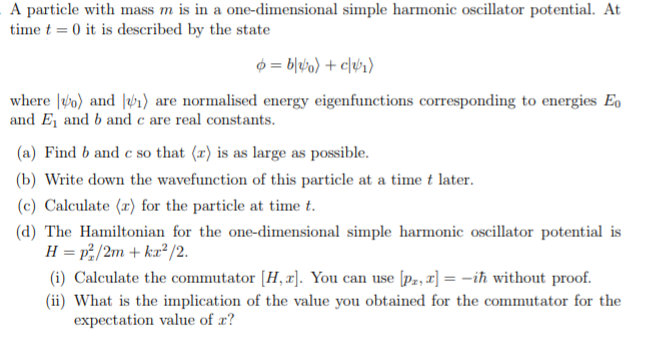A particle with mass m is in a one-dimensional simple harmonic oscillator potential. At time t = 0 it is described by the state where lo and l) are normalised energy eigenfunctions corresponding to energies E and Ey and b and c are real constants. (a) Find b and c so that (x) is as large as possible. b) Write down the wavefunction of this particle at a time t later c)Caleulate (x) for the particle at time t (d) The Hamiltonian for the one-dimensional simple harmonic oscillator potential is (i) Calculate the commutator [H,x]. You can use ip,-ih without proof. (ii) What is the implication of the value you obtained for the commutator for the expectation value of r?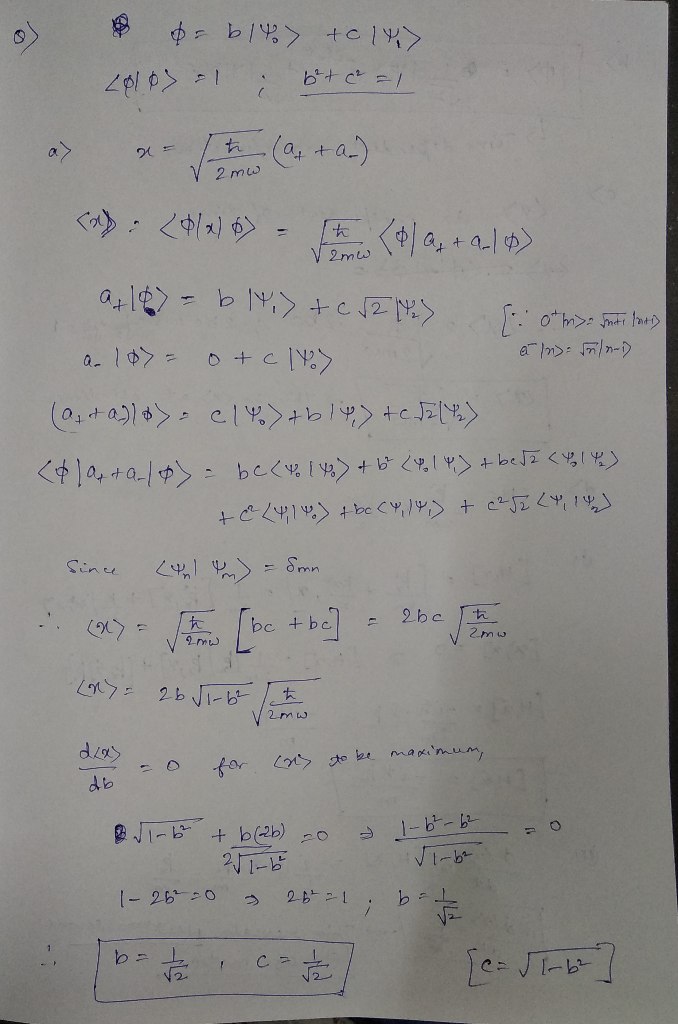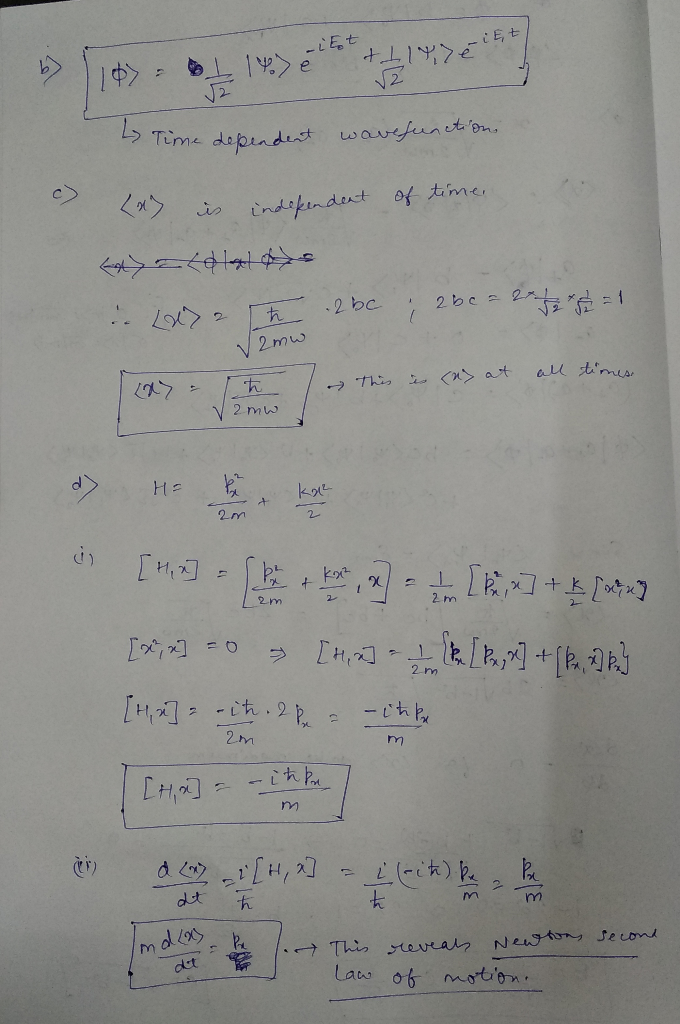Earn Coins

Coins can be redeemed for fabulous gifts.

Similar Homework Help Questions
• A particle with mass m is in a one dimensional simple harmonic oscillator potential. At timet0 it is described by the superposition state where Vo, 1 and Vz are normalised energy eigenfunctions of th...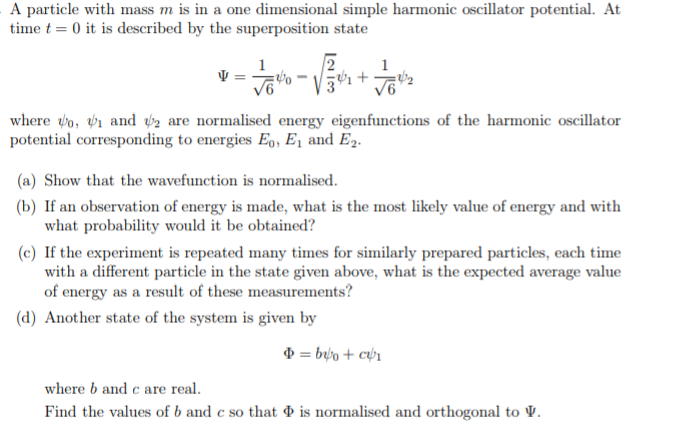A particle with mass m is in a one dimensional simple harmonic oscillator potential. At timet0 it is described by the superposition state where Vo, 1 and Vz are normalised energy eigenfunctions of the harmonic oscillator potential corresponding to energies Eo, E1 and E2 (a) Show that the wavefunction is normalised (b) If an observation of energy is made, what is the most likely value of energy and with what probability would it be obtained? (c) If the experiment is...

• Consider a particle with mass m described by the Hamilton operator for a one-dimensional harmonic oscillator...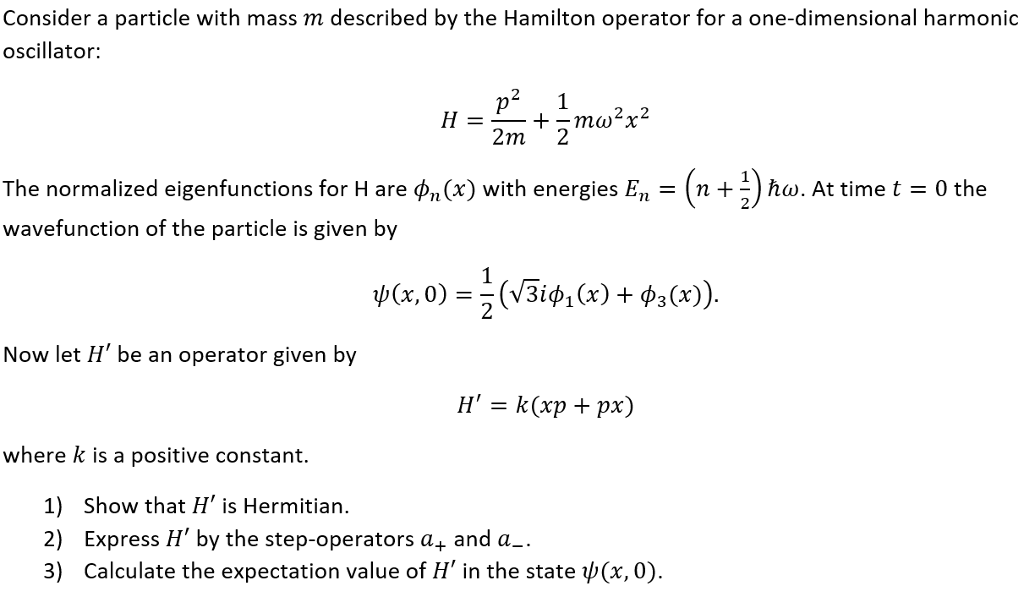Consider a particle with mass m described by the Hamilton operator for a one-dimensional harmonic oscillator 2 Zm 2 The normalized eigenfunctions for Hare φη (x) with energies E,,-(n + 2) ha. At time t-0 the wavefunction of the particle is given by у(x,0)- (V3іфі (x) + ф3(x)). Now let H' be an operator given by where k is a positive constant. 1) Show that H' is Hermitian. 2) Express H' by the step-operators a+ and a 3) Calculate the...

• A particle of mass m is bound by the spherically-symmetric three-dimensional harmonic- oscillator potential energy ,...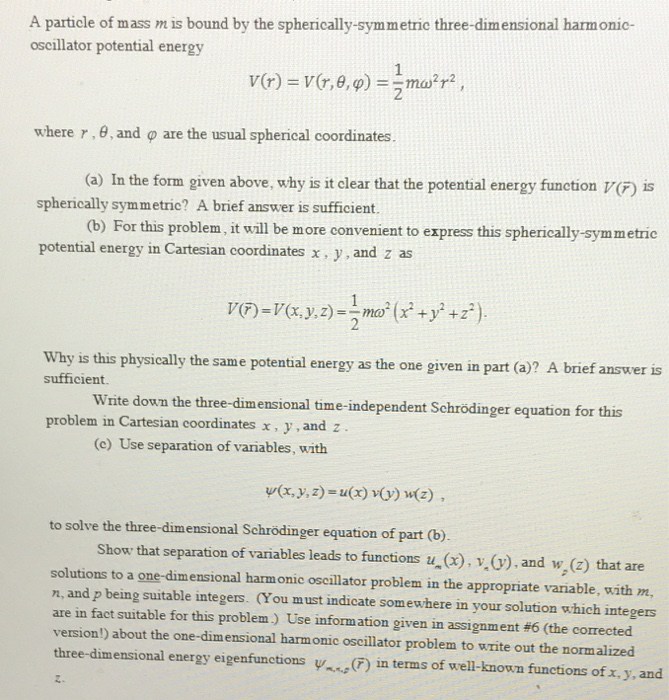A particle of mass m is bound by the spherically-symmetric three-dimensional harmonic- oscillator potential energy , and ф are the usual spherical coordinates. (a) In the form given above, why is it clear that the potential energy function V) is (b) For this problem, it will be more convenient to express this spherically-symmetric where r , spherically symmetric? A brief answer is sufficient. potential energy in Cartesian coordinates x, y, and z as physically the same potential energy as the...

• The most general wave function of a particle in the simple harmonic oscillator potential is: V(x,...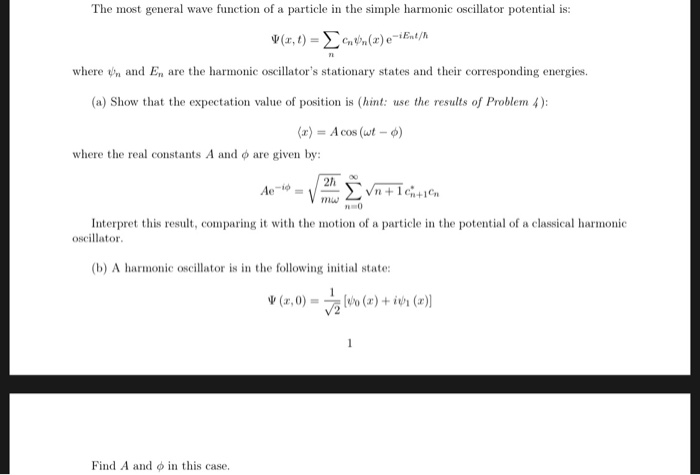The most general wave function of a particle in the simple harmonic oscillator potential is: V(x, t) = (x)e-1st/ where and E, are the harmonic oscillator's stationary states and their corresponding energies. (a) Show that the expectation value of position is (hint: use the results of Problem 4): (v) = A cos (wt - ) where the real constants A and o are given by: 1 2 Ae-id-1 " Entichtin Interpret this result, comparing it with the motion of a...

• At time t = 0 a particle in a Harmonic Oscillator potential is in the state...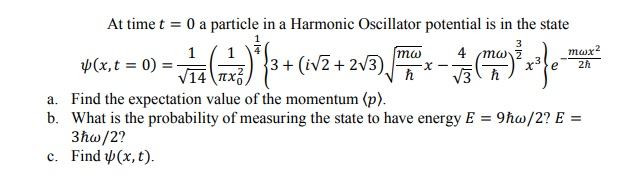At time t = 0 a particle in a Harmonic Oscillator potential is in the state plcx.e = 0) = va (23*43+(iv]+213) por mayorale * a. Find the expectation value of the momentum (p). b. What is the probability of measuring the state to have energy E = 9ħw/2? E = 3ħw/2? c. Find y(x, t).

• Estimate the ground-state energy of a one-dimensional simple harmonic oscillator using (50) = e-a-l as a...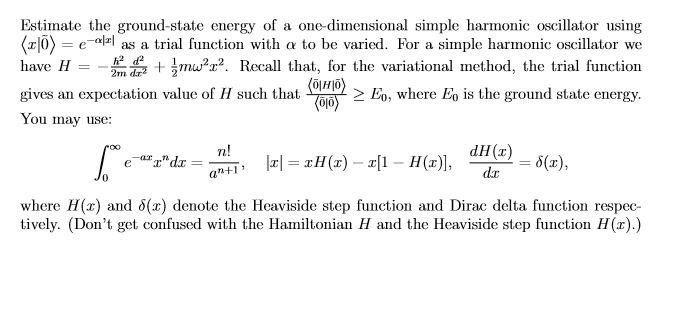Estimate the ground-state energy of a one-dimensional simple harmonic oscillator using (50) = e-a-l as a trial function with a to be varied. For a simple harmonic oscillator we have H + jmwºr? Recall that, for the variational method, the trial function (HO) gives an expectation value of H such that (016) > Eo, where Eo is the ground state energy. You may use: n! dH() ||= TH(c) – z[1 – H(r)], 8(2), dx S." arcade an+1 where (x) and...

• 3) A particle of mass m in the harmonic oscillator potential is initially described by a...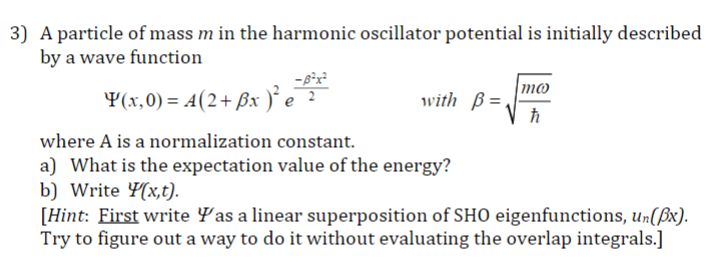3) A particle of mass m in the harmonic oscillator potential is initially described by a wave function with B- where A is a normalization constant a) What is the expectation value of the energy? b) Write Y[xt). [Hint: First write Ψas a linear superposition of SHO eigenfunctions, un(A) Try to figure out a way to do it without evaluating the overlap integrals.]

• please solve with explanations 3. (20 pts) A particle of mass m and charge q is in a one dimensional harmonic oscillato...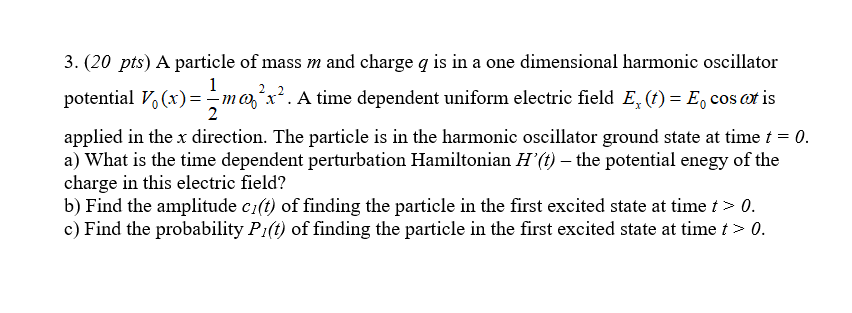please solve with explanations 3. (20 pts) A particle of mass m and charge q is in a one dimensional harmonic oscillator potential ()1ma'. A time dependent uniform electric field E, ()E, os eris 2 applied in the x direction. The particle is in the harmonic oscillator ground state at time a) What is the time dependent perturbation Hamiltonian H'(t) - the potential enegy of the charge in this electric field? b) Find the amplitude ci(t) of finding the particle...

• A particle of mass m in a harmonic oscillator potential #V(x)=(momega_0^2)/(2)x^2# has an initial #psi(x,0)=1/(sqrt2)[phi_0(x) + iphi_1(x)] # where #phi_n# are the normalized eigenstates for harmonic oscillator?

(a) Write down ψ(x,t) and |ψ(x,t)|^2 2. (For this part, you may leave the expression in terms of φ0 and φ1.) (b) Find the expectation value #<x># as a function of time t. Notice that it oscillates with time. What is the amplitude of the oscillation (in terms of m, ω0 and fundamental constants)? What is its angular frequency? (c) Find the expectation value (p) as a function of time. (d) Show that the probability distribution of a particle in...

• Consider a particle of mass m under the action of the one-dimensional harmonic oscillator potential. The Hamiltonian is...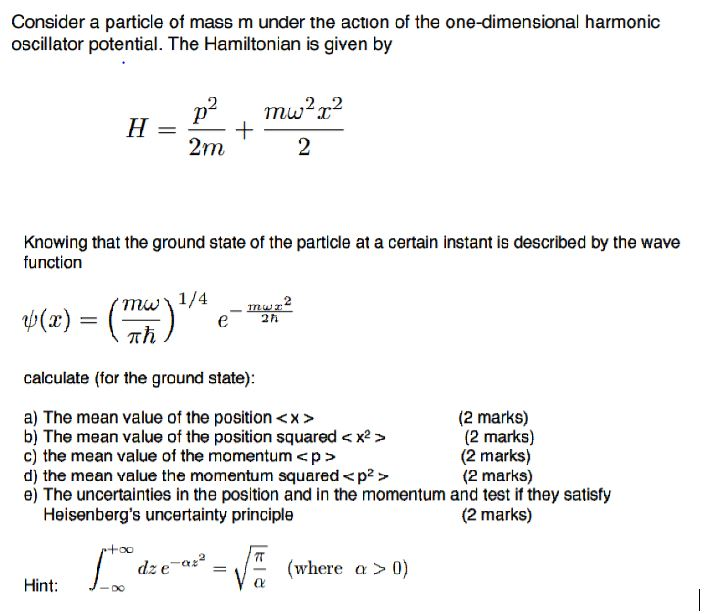Consider a particle of mass m under the action of the one-dimensional harmonic oscillator potential. The Hamiltonian is given by Knowing that the ground state of the particle at a certain instant is described by the wave function mw 1/4 _mw2 Th / calculate (for the ground state): a) The mean value of the position <x> (2 marks) b) The mean value of the position squared < x2 > (2 marks) c) the mean value of the momentum <p> (2...